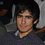# Yet another question about locating things!

This reminded me of yet another problem, but a more discreet one. It goes a bit like this.

A pair of lovers married yesterday, but in the craziness of the after party, they wake up in different houses (A house would be a point in the lattice grid Z*Z). The groom knows well that the wife is an anxious person, and will walk A streets North, B streets East and sleep there (A and B can be negative, and -4 north is 4 south for example), each day with constant A and B. "Woe is me!" He exclaims because he does not know where is she, and he does not know A nor B.

The groom is very fast so he can move from any house to another in a matter of seconds, but he can only check a house per day because he is a slow searcher. (That means, you can ask each day one house "Is the bride here?"). Can the groom, with complete certainty, catch the bride?Note by Diego Roque
5 years, 11 months ago

MarkdownAppears as
*italics* or _italics_ italics
**bold** or __bold__ bold
- bulleted- list
• bulleted
• list
1. numbered2. list
1. numbered
2. list
Note: you must add a full line of space before and after lists for them to show up correctly
paragraph 1paragraph 2

paragraph 1

paragraph 2

[example link](https://brilliant.org)example link
> This is a quote
This is a quote
    # I indented these lines
# 4 spaces, and now they show
# up as a code block.

print "hello world"
# I indented these lines
# 4 spaces, and now they show
# up as a code block.

print "hello world"
MathAppears as
Remember to wrap math in $ ... $ or $ ... $ to ensure proper formatting.
2 \times 3 $2 \times 3$
2^{34} $2^{34}$
a_{i-1} $a_{i-1}$
\frac{2}{3} $\frac{2}{3}$
\sqrt{2} $\sqrt{2}$
\sum_{i=1}^3 $\sum_{i=1}^3$
\sin \theta $\sin \theta$
\boxed{123} $\boxed{123}$

Sort by:

Simple! Once the bride reaches a corner, she cannot go any further. So the groom waits Z days and searches all the corners

- 5 years, 11 months ago

When Diego wrote "Z*Z", I think he meant $\mathbb{Z} \times \mathbb{Z}$, i.e. the set of ordered pairs of integers.

This set extends infinitely in all directions and doesn't have "corners" for the bride to get stuck in.

- 5 years, 11 months ago

Is there any time limit on how long the groom can take to catch the bride (other than being finite)? If not:

Let $(x,y)$ be the initial house the bride is at, Then, on the $n$-th day, she is at $(x+An,y+Bn)$.

We know that $\mathbb{N}_0$ and $\mathbb{Z}^4$ have the same cardinality. So, let $f : \mathbb{N}_0 \to \mathbb{Z}^4$ be any bijection.

On the $n$-th day, compute $(x',y',A',B') = f(n)$. Then, have the groom search $(x'+A'n,y',B'n)$.

In other words, on the $n$-th day, the groom guesses that the bride started at the point $(x',y')$ and moves $(A',B')$ each day, and then searches the house the bride would be in based on that guess.

On the $f^{-1}(x,y,A,B)$-th day, the groom guessed correctly, and therefore found the bride.

Note that this could take an arbitrarily long, but finite time.

- 5 years, 11 months ago

This problem as well as the pirate ship problem have the following general strategy:

1. Find a formula for the position of the target at any time in terms of some set of initial parameters.

2. At each time, make a different guess for the values of those parameters, and go to the corresponding location.

3. Make sure that you will guess correctly after a finite amount of time.

- 5 years, 11 months ago